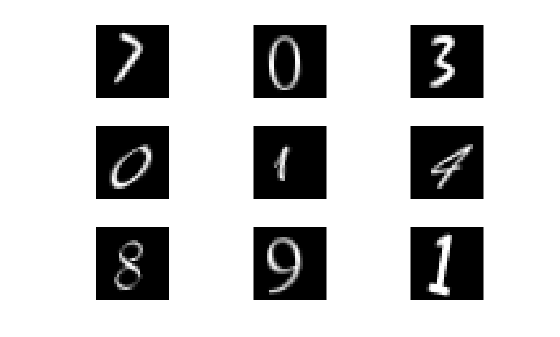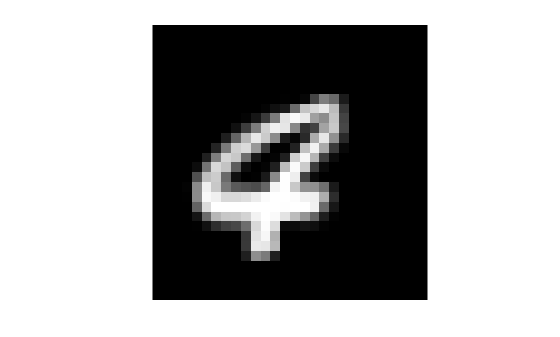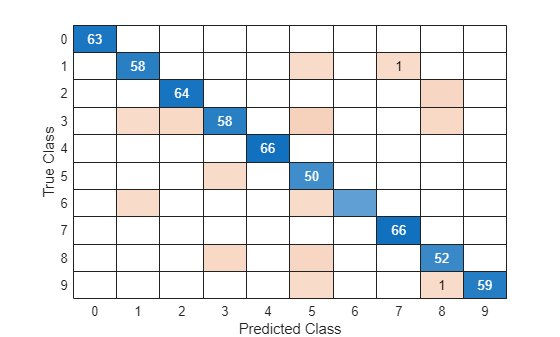Documentation

Code Generation for Image Classification

This example shows how to generate C code from a MATLAB function that classifies images of digits using a trained classification model. This example demonstrates an alternative workflow to Digit Classification Using HOG Features (Computer Vision Toolbox). However, to support code generation in that example, you can follow the code generation steps in this example.

Automated image classification is an ubiquitous tool. For example, a trained classifier can be deployed to a drone to automatically identify anomalies on land in captured footage, or to a machine that scans handwritten zip codes on letters. In the latter example, after the machine finds the ZIP code and stores individual images of digits, the deployed classifier must guess which digits are in the images to reconstruct the ZIP code.

This example shows how to train and optimize a multiclass error-correcting output codes (ECOC) classification model to classify digits based on pixel intensities in raster images. The ECOC model contains binary support vector machine (SVM) learners. Then, this example shows how to generate C code that uses the trained model to classify new images. The data are synthetic images of warped digits of various fonts, which simulates handwritten digits.

Set Up Your C Compiler

To generate C/C++ code, you must have access to a C/C++ compiler that is configured properly. MATLAB Coder locates and uses a supported, installed compiler. You can use mex -setup to view and change the default compiler. For more details, see Change Default Compiler (MATLAB).

Assumptions and Limitations

To generate C code, MATLAB Coder:

• Requires a properly configured compiler.

• Requires supported functions to be in a MATLAB function that you define. For the basic workflow, see Introduction to Code Generation.

• Forbids objects as input arguments of the defined function.

Concerning the last limitation, consider that:

• Trained classification models are objects

• MATLAB Coder supports predict to classify observations using trained models, but does not support fitting the model

To work around the code generation limitations for classification, train the classification model using MATLAB, then pass the resulting model object to saveLearnerForCoder. The saveLearnerForCoder function removes some properties that are not required for prediction, and then saves the trained model to disk as a structure array. Like the model, the structure array contains the information used to classify new observations.

After saving the model to disk, load the model in the MATLAB function by using loadLearnerForCoder. The loadLearnerForCoder function loads the saved structure array, and then reconstructs the model object. In the MATLAB function, to classify the observations, you can pass the model and predictor data set, which can be an input argument of the function, to predict.

Code Generation for Classification Workflow

Before deploying an image classifier onto a device:

1. Obtain a sufficient amount of labeled images.

2. Decide which features to extract from the images.

3. Train and optimize a classification model. This step includes choosing an appropriate algorithm and tuning hyperparameters, that is, model parameters not fit during training.

4. Save the model to disk by using saveLearnerForCoder.

5. Define a function for classifying new images. The function must load the model by using loadLearnerForCoder, and can return labels, such as classification scores.

6. Set up your C compiler.

7. Decide the environment in which to execute the generated code.

8. Generate C code for the function.

Load the digitimages data set.

images is a 28-by-28-by-3000 array of uint16 integers. Each page is a raster image of a digit. Each element is a pixel intensity. Corresponding labels are in the 3000-by-1 numeric vector Y. For more details, enter Description at the command line.

Store the number of observations and number of predictor variables. Create a data partition that specifies to hold out 20% of the data. Extract training and test set indices from the data partition.

rng(1) % For reproducibility
n = size(images,3);
p = numel(images(:,:,1));
cvp = cvpartition(n,'Holdout',0.20);
idxTrn = training(cvp);
idxTest = test(cvp);

Display nine random images from the data.

figure
for j = 1:9
subplot(3,3,j)
selectImage = datasample(images,1,3);
imshow(selectImage,[])
endRescale Data

Because raw pixel intensities vary widely, you should normalize their values before training a classification model. Rescale the pixel intensities so that they range in the interval [0,1]. That is, suppose ${p}_{ij}$ is pixel intensity $j$ within image $i$. For image $i$, rescale all of its pixel intensities using this formula:

${\underset{}{\overset{ˆ}{p}}}_{ij}=\frac{{p}_{ij}-\underset{j}{\mathrm{min}}\left({p}_{ij}\right)}{\underset{j}{\mathrm{max}}\left({p}_{ij}\right)-\underset{j}{\mathrm{min}}\left({p}_{ij}\right)}.$

X = double(images);
for i = 1:n
minX = min(min(X(:,:,i)));
maxX = max(max(X(:,:,i)));
X(:,:,i) = (X(:,:,i) - minX)/(maxX - minX);
end

Alternatively, if you have an Image Processing Toolbox™ license, then you can efficiently rescale pixel intensities of images to [0,1] by using mat2gray. For more details, see mat2gray.

Reshape Data

For code generation, the predictor data for training must be in a table of numeric variables or a numeric matrix.

Reshape the data to a matrix such that predictor variables (pixel intensities) correspond to columns, and images (observations) to rows. Because reshape takes elements column-wise, you must transpose its result.

X = reshape(X,[p,n])';

To ensure that preprocessing the data maintains the image, plot the first observation in X.

figure
imshow(reshape(X(1,:),sqrt(p)*[1 1]),[],'InitialMagnification','fit')Extract Features

Computer Vision Toolbox™ offers several feature-extraction techniques for images. One such technique is the extraction of histogram of oriented gradient (HOG) features. To learn how to train an ECOC model using HOG features, see Digit Classification Using HOG Features (Computer Vision Toolbox). For details on other supported techniques, see Local Feature Detection and Extraction (Computer Vision Toolbox). This example uses the rescaled pixel intensities as predictor variables.

Train and Optimize Classification Model

Linear SVM models are often applied to image data sets for classification. However, SVM are binary classifiers, and there are 10 possible classes in the data set.

You can create a multiclass model of multiple binary SVM learners using fitcecoc. fitcecoc combines multiple binary learners using a coding design. By default, fitcecoc applies the one-versus-one design, which specifies training binary learners based on observations from all combinations of pairs of classes. For example, in a problem with 10 classes, fitcecoc must train 45 binary SVM models.

In general, when you train a classification model, you should tune the hyperparameters until you achieve a satisfactory generalization error. That is, you should cross-validate models for particular sets of hyperparameters, and then compare the out-of-fold misclassification rates.

You can choose your own sets of hyperparameter values, or you can specify to implement Bayesian optimization. (For general details on Bayesian optimization, see Bayesian Optimization Workflow.) This example performs cross-validation over a chosen grid of values.

To cross-validate an ECOC model of SVM binary learners based on the training observations, use 5-fold cross-validation. Although the predictor values have the same range, to avoid numerical difficulties during training, standardize the predictors. Also, optimize the ECOC coding design and the SVM box constraint. Use all combinations of these values:

• For the ECOC coding design, use one-versus-one and one-versus-all.

• For the SVM box constraint, use three logarithmically-spaced values from 0.1 to 100 each.

For all models, store the 5-fold cross-validated misclassification rates.

coding = {'onevsone' 'onevsall'};
boxconstraint = logspace(-1,2,3);
cvLoss = nan(numel(coding),numel(boxconstraint)); % For preallocation

for i = 1:numel(coding)
for j = 1:numel(boxconstraint)
t = templateSVM('BoxConstraint',boxconstraint(j),'Standardize',true);
CVMdl = fitcecoc(X(idxTrn,:),Y(idxTrn),'Learners',t,'KFold',5,...
'Coding',coding{i});
cvLoss(i,j) = kfoldLoss(CVMdl);
fprintf('cvLoss = %f for model using %s coding and box constraint=%f\n',...
cvLoss(i,j),coding{i},boxconstraint(j))
end
end
cvLoss = 0.052083 for model using onevsone coding and box constraint=0.100000
cvLoss = 0.055000 for model using onevsone coding and box constraint=3.162278
cvLoss = 0.050000 for model using onevsone coding and box constraint=100.000000
cvLoss = 0.116667 for model using onevsall coding and box constraint=0.100000
cvLoss = 0.123750 for model using onevsall coding and box constraint=3.162278
cvLoss = 0.125000 for model using onevsall coding and box constraint=100.000000

Determine the hyperparameter indices that yield the minimal misclassification rate. Train an ECOC model using the training data. Standardize the training data and supply the observed, optimal hyperparameter combination.

minCVLoss = min(cvLoss(:))
minCVLoss = 0.0500
linIdx = find(cvLoss == minCVLoss);
[bestI,bestJ] = ind2sub(size(cvLoss),linIdx);
bestCoding = coding{bestI}
bestCoding =
'onevsone'
bestBoxConstraint = boxconstraint(bestJ)
bestBoxConstraint = 100
t = templateSVM('BoxConstraint',bestBoxConstraint,'Standardize',true);
Mdl = fitcecoc(X(idxTrn,:),Y(idxTrn),'Learners',t,'Coding',bestCoding);

Construct a confusion matrix for the test set images.

testImages = X(idxTest,:);
testLabels = predict(Mdl,testImages);
confusionMatrix = confusionchart(Y(idxTest),testLabels);Diagonal and off-diagonal elements correspond to correctly and incorrectly classified observations, respectively. Mdl seems to correctly classify most images.

If you are satisfied with the performance of Mdl, then you can proceed to generate code for prediction. Otherwise, you can continue adjusting hyperparameters. For example, you can try training the SVM learners using different kernel functions.

Save Classification Model to Disk

Mdl is a predictive classification model, but you must prepare it for code generation. Save Mdl to your present working directory using saveLearnerForCoder.

saveLearnerForCoder(Mdl,'DigitImagesECOC')

saveLearnerForCoder compacts Mdl, converts it to a structure array, and saves it in the MAT-file DigitImagesECOC.mat.

Define Prediction Function for Code Generation

Define an entry-point function named predictDigitECOC.m that does the following:

• Include the code generation directive %#codegen somewhere in the function.

• Accept image data commensurate with X.

• Return predicted labels.

type predictDigitECOC.m % Display contents of predictDigitECOC.m file
function label = predictDigitECOC(X) %#codegen
%PREDICTDIGITECOC Classify digit in image using ECOC Model
%   PREDICTDIGITECOC classifies the 28-by-28 images in the rows of X using
%   the compact ECOC model in the file DigitImagesECOC.mat, and then
%   returns class labels in label.
label = predict(CompactMdl,X);
end

Note: If you click the button located in the upper-right section of this page and open this example in MATLAB®, then MATLAB® opens the example folder. This folder includes the entry-point function file.

Verify that the prediction function returns the same test set labels as predict.

pfLabels = predictDigitECOC(testImages);
verifyPF = isequal(pfLabels,testLabels)
verifyPF = logical
1

isequal returns logical 1 (true), which means all the inputs are equal. The predictDigitECOC yields the expected results.

Decide Which Environment to Execute Generated Code

Generated code can run:

• Inside the MATLAB environment as a C-MEX file

• Outside the MATLAB environment as a standalone executable

• Outside the MATLAB environment as a shared utility linked to another standalone executable

This example generates a MEX file to be run in the MATLAB environment. Generating such a MEX file allows you to test the generated code using MATLAB tools before deploying the function outside the MATLAB environment. In the MEX function, you can include code for verification, but not for code generation, by declaring the commands as extrinsic using coder.extrinsic. Extrinsic commands can include functions that do not have code generation support. All extrinsic commands in the MEX function run in MATLAB, but codegen does not generate code for them.

If you plan to deploy the code outside the MATLAB environment, then you must generate a standalone executable. One way to specify your compiler choice is by using the -config option of codegen. For example, to generate a static C executable, specify -config:exe when you call codegen. For more details on setting code generation options, see the -config option of codegen.

Compile MATLAB Function to MEX File

Compile predictDigitECOC.m to a MEX file using codegen. Specify these options:

• -report — Generates a compilation report that identifies the original MATLAB code and the associated files that codegen creates during code generation.

• -args — MATLAB Coder requires that you specify the properties of all the function input arguments. One way to do this is to provide codegen with an example of input values. Consequently, MATLAB Coder infers the properties from the example values. Specify the test set images commensurate with X.

codegen predictDigitECOC -report -args {testImages}
Code generation successful: View report

codegen successfully generated the code for the prediction function. You can view the report by clicking the link at the command line. If code generation is unsuccessful, then the report can help you debug.

codegen creates the directory pwd/codegen/mex/predictDigitECOC, where pwd is your present working directory. In the child directory, codegen generates, among other things, the MEX-file predictDigitECOC_mex.mexw64.

Verify that the MEX file returns the same labels as predict.

mexLabels = predictDigitECOC_mex(testImages);
verifyMEX = isequal(mexLabels,testLabels)
verifyMEX = logical
1

isequal returns logical 1 (true), meaning that the MEX-file yields the expected results.